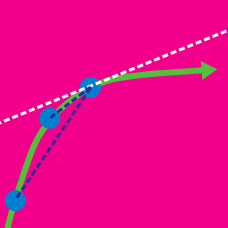Calculus

# Tangent Line at a Point

Which of the following is the tangent line to the curve $f(x)=2x^3-8x^2$ at the point $(1,-6)?$

Find the equation of the tangent line to the curve $x^2y-x=4y^3-108$ for $x=0.$

What is the equation of the line that passes through the point $(3,24)$ and is perpendicular to the tangent line of the curve $y=x^2+5x$ at that point?

Given the curve $x=t^3, y=4t^2+9,$ what is the $y$-intercept of the tangent line to the curve at $t=1 ?$

What is the $y$-intercept of the tangent line to the curve $y=e^{x-4}$ at the point $(5,e)?$

×